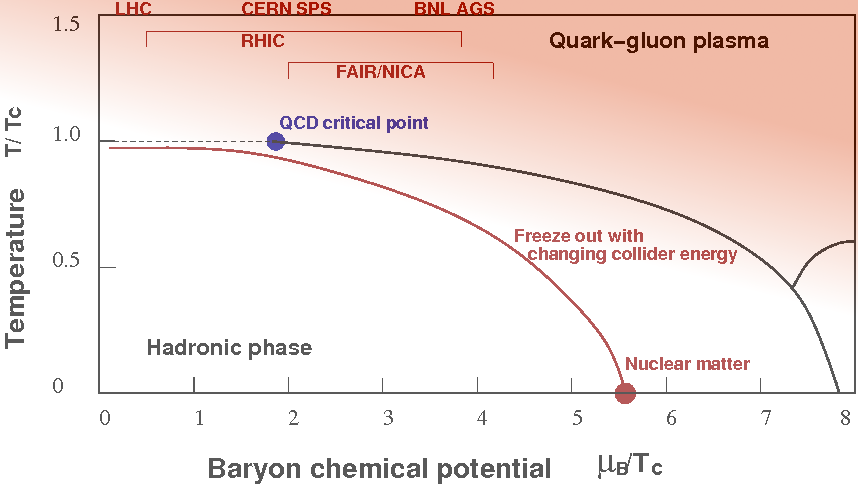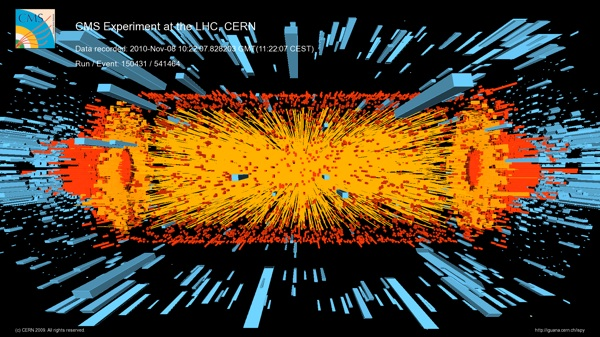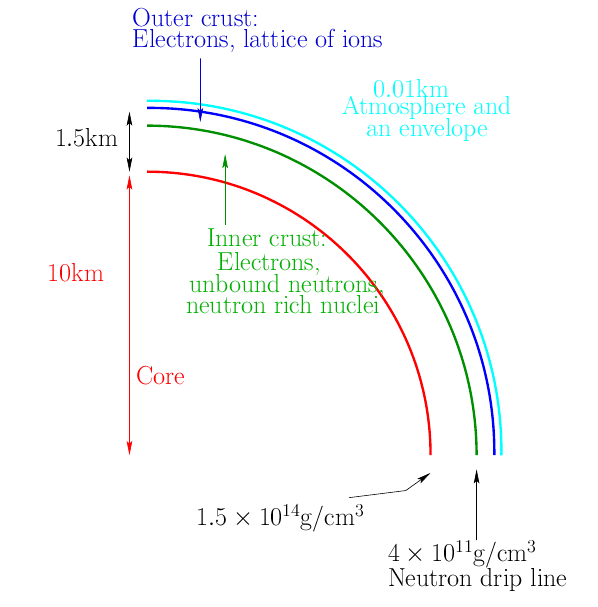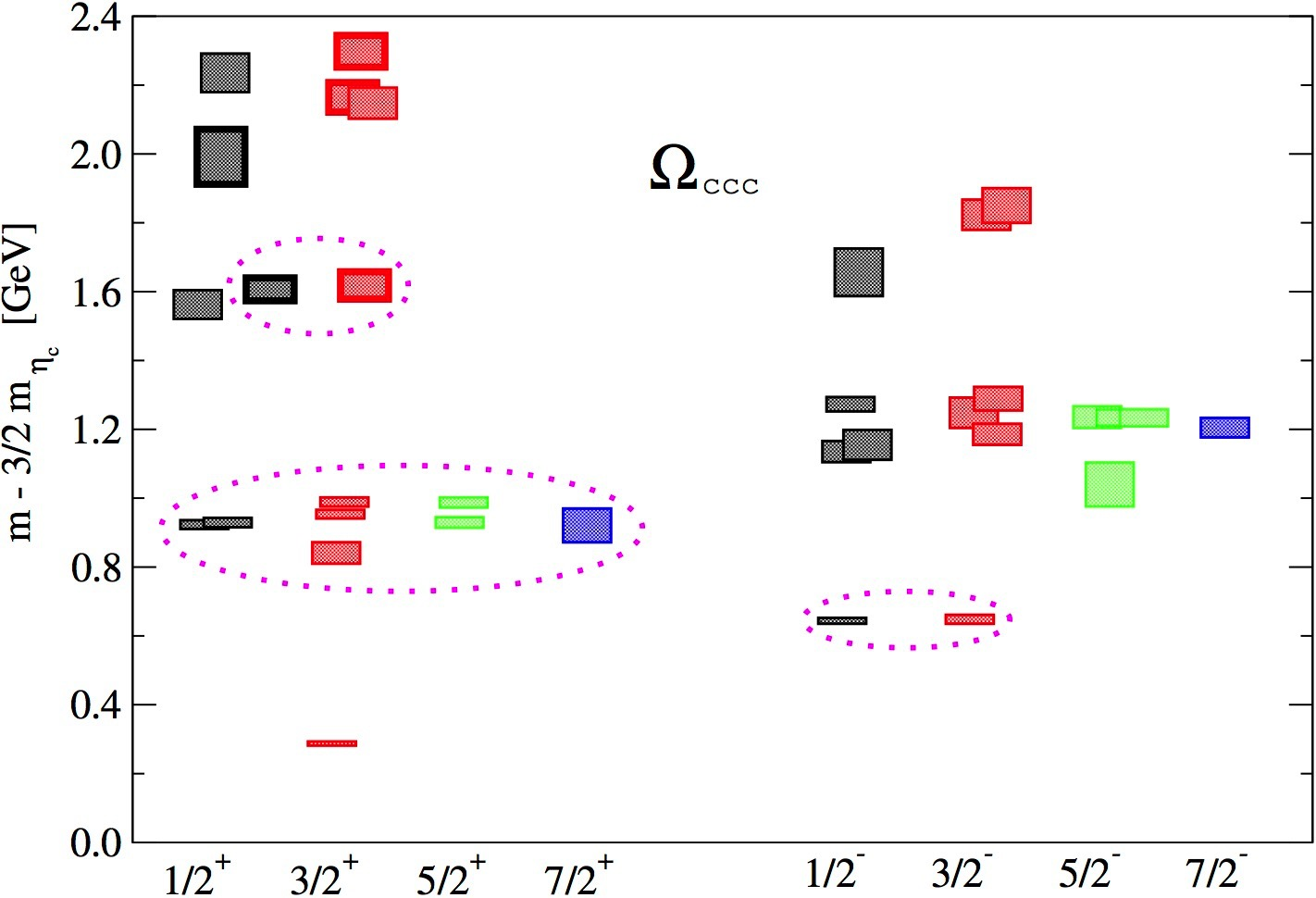# Quantum Chromo-Dynamics

Rajeev S. Bhalerao, Saumen Datta, Rajiv V. Gavai, Sourendu Gupta, Nilmani Mathur, and Rishi Sharma#### QCD phase diagram

At temperatures $$T$$ and/or quark number chemical potential $$\mu$$ large compared to the typical energy scale of strong interactions, $$\Lambda_{\rm{QCD}}$$, we expect quarks and gluons to be deconfined. At low net baryon densities, the transition to this phase can be studied in a theoretically rigorous manner by using the techniques of lattice QCD. For $$\mu=0$$ we now understand that the transition to a deconfined hot plasma of quarks and gluons [a quark gluon plasma or the QGP] is a crossover. We also expect on theoretical grounds that at the transition to a deconfined phase at low $$T$$ and high $$\mu$$ is first order. This curve of first order transitions should terminate at some finite $$T$$ and $$\mu$$ at a critical point. Members of our group are using sophisticated techniques to calculate the location of the critical point in the $$T$$, $$\mu$$ plane. We are also investigating various features of the phase diagram using a combination of numerical and analytical tools.#### Heavy Ion Collisions

The Quark Gluon Plasma (QGP) is experimentally studied in heavy ion collisions at RHIC and at the LHC. Hydrodynamic modelling of these collisions, an activity intensely pursued by members, suggests that a thermally equilibrated plasma is created very shortly after the collision and this plasma has a viscosity over entropy ratio small compared to most of the fluids encountered in daily life. One method of probing this hydrodynamic medium is by studying how it affects the propagation of heavy or energetic particles: a subject being pursued here.

Photo Courtesy: CERN#### Neutron stars

On the other extreme end, at $$\mu$$ much larger than $$\Lambda_{\rm{QCD}}$$ but small $$T$$, deconfined matter will be a cold plasma of quarks and gluons, where the quarks will exist in some form of a color superconducting phase. This form of matter can exist in neutron stars, and members of the group are trying to understand how their presence can affect the structure of neutron stars and their dynamics.#### Hadronic Physics

The properties of hadrons can be calculated from QCD using numerical Monte Carlo techniques. Members of our group are investigating the spectrum and form factors of hadrons. In particular, we are engaged in investigation of hadrons with one or more heavy (charm/bottom) quarks. These calculations provide predictions for ongoing and upcoming experiments like LHC, BELLE, BES, and PANDA, where many such hadrons are expected to be discovered. The form factors are important ingredients in experimental determination of CKM matrix elements, which are central to our understanding of matter-antimatter asymmetry.